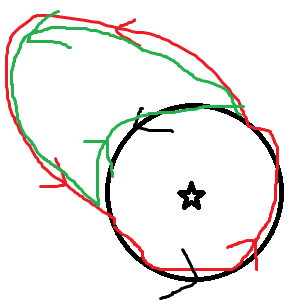# Cauchy's integral formula

• I
Hi.
I am working my way through some complex analysis notes(from a physics course). I have just covered Cauchy's theorem which basically states that the integral over a closed contour of an analytic function is zero. this is then used to show that contours of analytic functions can be deformed and the integrals still give the same answer.

My problem begins when Cauchy's integral formula is introduced and it is used to compute an integral of 1/z around a unit circle. The notes then state that an arbitrary curve can be deformed to a circle using Cauchy's theorem but this theorem is only valid for analytic functions and 1/z is not analytic at the origin. So how can arbitrary curves be deformed ?
Thanks

FactChecker
Gold Member
If the curve does not wind around z=0, then Cauchy's Theorem says that it can be shrunk down to nothing and the integral is 0. If it does wind around z=0 (circling around and then circling back does not count), then Cauchy's Theorem says that it can be shrunk down to a tiny circle around z=0 which winds around it the same number of times. That does NOT give an integral of zero. It is not hard to determine the integral of that tiny circle and the result is called the "residue" at z=0. (If it winds around once in the counter-clockwise direction, the integral is ##2\pi i## (see https://en.wikipedia.org/wiki/Cauchy%27s_integral_theorem#Discussion).This is a very important part of complex analysis, called the Residue Theorem (see https://en.wikipedia.org/wiki/Residue_theorem).

Last edited:
I havn't got to the Residue theorem yet but deforming contours around a function that is not analytic at a point inside the contour using only Cauchy's theorem doesn't seem right. It seems to be using ideas from further on in the course possibly

FactChecker
Gold Member
No, it doesn't rely on anything more advanced. The deformation of the path is ok as long as it is not moved through the z=0 point. In general, as long as the path is moved smoothly through a region where the function is analytic, the integral does not change. That is why you can shrink the path down to only a tiny circle around z=0. The function is analytic everywhere else. But you can not move the path through the z=0 point at all.

•mathwonk
In general, as long as the path is moved smoothly through a region where the function is analytic, the integral does not change. .
But 1/z is not analytic at the origin

mfb
Mentor
But you don't change the path at the origin. You change it in a region where the function is analytic.

I made a bad paint sketch. The star is the singularity at z=0. Imagine you want to evaluate the integral around the red path. You can express it as sum of the integral around the black circle (which you know) and the green path. The green path is an integral of a function which is analytic everywhere on the closed path and inside: Its integral is zero. Therefore the red and the black paths have the same integral.#### Attachments

•dyn, mathwonk and FactChecker
Thank you. Your sketch did the job ! I can totally see it now. Thanks